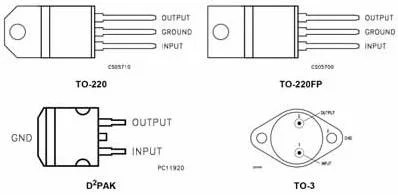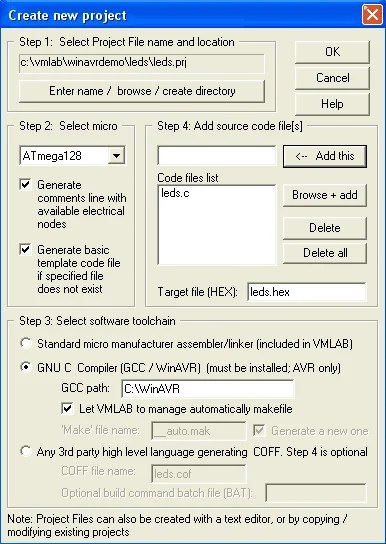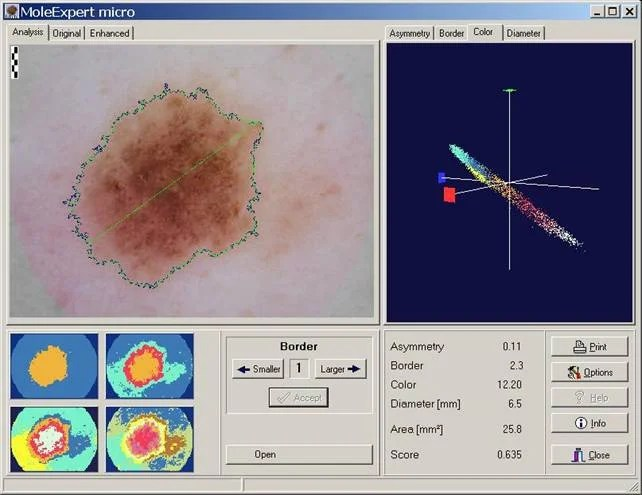## 7805 voltage regulator for your designThis is most common voltage regulator that is still used in embedded designs. LM7805 voltage regulator is a linear regulator made by several manufacturers like Fairchild, or ST Microelectronics. They can come in several types of packages. For output current up to 1A there may be two types of packages: TO-220 (vertical) and D-PAK (horizontal). With proper heat sink these LM78xx types can handle even more than 1A current. They also have Thermal overload protection, Short circuit protection. If your design wonâ€™t exceed 0.1A current you may chose regulator LM78L05 with smaller packages and lower maximum current up to 0.1A. They come in three main types of packages SO-8, SOT-89 and TO-92 Bellow is a table OF all LM78XX regulators and their output Voltages and input voltage ranges. Part Output (V) Input range (V) LM7805 5 7â€“25 LM7806 6 8â€“25 LM7808 8 10.5â€“25 LM7809 9 11.5â€“25 LM7810 10 12.5â€“25 LM7812 12 14.5â€“30 LM7815 15 17.5â€“30 LM7818 18 21â€“33 LM7824 24 27â€“38 Typical Connection is very simple: Couple decoupling capacitors (between 10 uF and 47 uF) are required on the input (V-IN) and output (V-OUT) connected to ground. There are negative voltage regulators that work the same way. They are marked…

## Using VMLAB as virtual oscilloscopeVMLAB is one of well known simulators. This is software simulator of AVR and ST62 microcontrollers. You may download free version of it from https://www.amctools.com. The simulation is far away from real time, but All timings are tied to real world values. VMLAB is designed to work as project. This is a special language (script like), where circuit is described – where are connections between hardware and microcontroller defined. Few prebuilt examples you may find in folders C:\VMLAB\AVR_demo and C:\VMLAB\ WinAVRdemo (If your installation is in C:\VMLAB\ folder). VMLAB is rich in its hardware support: Resistor, Grounded capacitor, Interactive switch / key, LED diode, Pulsed voltage source, Sine wave voltage source, Slider dependent voltage source (interactive), Non-ruturn-to-zero (NRZ) generator (interactive), Operational amplifier, Comparator, 2 inputs NAND gate, 8 bits D to A converter, RS232 based TTY (interactive), LCD module, I2C monitor (interactive), Interactive keypad 4×4 Multiprocess-dedicated: External Input, External Output. So you can do wide range of simulations. VMLAB also has a powerful scope where you can watch voltages on pins or even some internal microcontroller resistor values like ACO, TIMOVF signals. Let’s make simple project using VMLAB tool. Start VMLAB And create new project by selecting Project-New. Select project…

## MoleExpert micro softwareThe MoleExpert software is a product is based on experiences of many years with the automated analysis of pigmented skin lesions. Essential requirement with this software project was the usefulness of the software with the most different photograph systems. Qualitatively high-quality, evenly and well illuminated top illumination-microscopic pictures of the lesions is the most essential condition for the operability of this software. MoleExpert micro was developed for the support of diagnostic identification. The system spends no diagnosis for this reason, but supplies as results of measurement data to asymmetry, for the delimitation of the lesion, to the color and the size. These parameters of the ABCD rule are recognized for some years as important dermatoscopic parameters. According to a particular algorithm adapted on the image analysis, the four ABCD values are combined into a total core, which can take values between zero to unify. With lesions with high Score, it acts with higher probability around a Melanoma, than with lesions with low Score. Download demo version from here: MoleExpert micro

## Binary watch with LED matrix display

In this video, the guy managed to make a binary wristwatch with an LED display. All settings are accessible through a scrolling menu system on its 3×4 LED matrix. Watch also include voltage meter, binary counter, club mode, and time display.

## Frequency response of discrete systemIn previous post we discussed about impulse response. Impulse response h(n) is digital system response in time domain. But there is another characterization of discrete system – frequency response H(ejω). Frequency response can be calculated form impulse response by formula: This means frequency response is systems transfer coefficient to every frequency value. Frequency response is a complex function. So this can be evaluated as:

## Digital system equationBack to DSP thread. Lets talk about digital system equation. Every digital system can be described using this equation. The output of digital filter generally consist of previous inputs and previous outputs. y(n-k) is considered as previous outputs; x(n-p) – previous inputs; ak and b­p – coefficients; This equation is convenient to define discrete system and extract various characteristics. Number N defines discrete filter tap (Tap – A FIR “tap” is simply a coefficient/delay pair). Lets say we have digital filter made of one tap. y(n)=a·y(n-1)+b·x(n) First we calculate filter response function – h(n): Response function is calculated from system reaction to discrete impulse ´(n). So assume that x(n)= ´(n) and y(n)=h(n). Initial conditions: y(-1)=h(-1)=0. Then: h(n)=0 when n<0; h(0)=a·h(-1)+b·´(n)=b; h(1)=a·h(0)+b·´(1)=ab; ……. h(n)= ban where n>0 Lets say we have a=0.7; b=1; Then we get discrete system characteristics: This equation in example describes IIR (Infinite Impulse Response) discrete systems, because there is a feedback element a·y(n-1). There is another type of discrete filter – FIR (Finite Impulse Response) Finite response filter equation is much simpler: As you may noticed from equation – IIR filter have infinite number of response impulses while FIR filter have a finite number of impulses. IIR…

## AVR microcontroller interrupts using WINAVR